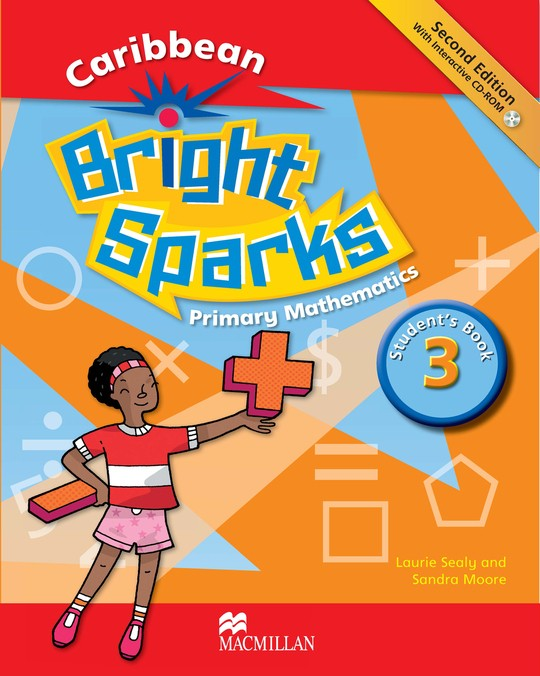Macmillan EducationLaurie Sealy
Bright Sparks Level 3 Student's Book
US\$ 13.51
The publisher has enabled DRM protection, which means that you need to use the BookFusion iOS, Android or Web app to read this eBook. This eBook cannot be used outside of the BookFusion platform.
Description
Contents
Reviews

Bright Sparks is a Mathematics course for pupils in Caribbean primary schools. It has been written in line with the latest primary curriculum requirements and is based on current research in Mathematics education.Young children learn Mathematics best when they use their senses in varied activities, experimenting and discovering. The Bright Sparks series is intended to supplement and reinforce the learning first gained through practical activities.Key features include:• Examples and word problems suited to the age level• Challenge boxes push students’ learning and are ideal for quick learners• Remember boxes highlight important facts or skills• Hint or Think boxes stimulate thinking and develop reasoning• Partner activities encourage students to learn with and from their peers• Discuss encourages students to explain their thinking and strategies• Review provides reminders and practice for all learners• Mathematical language boxes develop mathematical vocabulary• IT icons show where IT support can be found on the interactive CD-ROMs, and for calculator practice• Enrichment pages move beyond the syllabus and make connections to familiar, real-life situations• Unit Summaries highlight the key learning points in each section• Unit Checks test students’ learning of each topic• Assessments are provided in a variety of different formsPlease note that this is an eBook version of this title and can NOT be printed. For more information about eBooks, including how to download the software you’ll need, see our FAQs page.

Language
English
ISBN
9780230465145
Cover
Title
Contents
Scope and sequence
How to use Bright Sparks – for students
Introduction – for teachers
How to use this CD-ROM
Unit 1: Patterns and numbers
Counting in twos
Counting in threes
Counting in 5s
Counting in 10s
Number patterns
Continuing sequences
More number patterns
Comparing numbers
Equal numbers
Comparing: practical situations
Sets
Odd and even numbers
Counting on: practical situations
Working backwards
Writing number words
Ordinal numbers
Unit 1 Check and summary
Assessment 1
Unit 2: Number
Place value
Value
Value – expanded numbers
Expanded numbers practice
Rounding
Rounding cents to the nearest ten
Rounding to the nearest hundred
Rounding to the nearest dollar
Making ten
Unit 2 Check and summary
Assessment 2
Unit 3: Operations – addition and subtraction
Addition – regrouping tens in 3-digit numbers
Subtraction review
Algebra – missing numbers
Subtraction with 2-digit numbers
Practise regrouping
Subtraction with regrouping
Subtraction with hundreds – regrouping tens
Subtraction practice
Fact families for + and -
Problem solving
Unit 3 Check and summary
Assessment 3
Unit 4: Operations – multiplication
Calculator fun
Multiplication – times
2-digit multiplication
Multiplication with regrouping
Multiplying by 3
Multiplying by 4
Multiplying – regrouping to hundreds
Multiplying by 5
Multiplying by 10
Multiplying using arrays
Multiplying 6, 7, 8 and 9 (Enrichment)
Problem solving
Unit 4 Check and summary
Unit 5: Operations – division
Division as sharing
Division as grouping
Dividing a 2-digit number by 2
Dividing with a remainder
Dividing by 2 – regrouping tens
Problem solving
More regrouping tens
Dividing by 3
Dividing a 2-digit number by 3
Mixed problems
Dividing by 3 with a remainder
Dividing by 4
Dividing by 5
Dividing by 4 and 5 using columns
Dividing by 4 and 5 with a remainder
Fact families for × and ÷
Unit 5 Check and summary
Assessment 4
Unit 6: Money
Money overview
Finding the total – coins
Drawing coins
Finding the total – notes
Writing sums of money
Drawing change and totals
Shopping practice
Spending money
Making change from \$10 or \$20
Practice making change
Larger notes
Rounding cents to the nearest dollar
Calculator activities – money
Unit 6 Check and summary
Assessment 5
Unit 7: Geometry
Congruence
Lines of symmetry
Drawing symmetrical shapes
Lines and angles
Right angles
Plane shapes (2D)
Identifying plane shapes (2D)
Solid shapes (3D)
Solid shapes – cylinders and spheres
Solid shapes – properties
Solid shapes in the environment
Unit 7 Check and summary
Unit 8: Measurement
Distance
Practical measurement
Estimating and measuring distance
Practice with distance
Capacity
Practice with capacity
Mass
Practical activity: mass
Customary and metric units (Enrichment)
Temperature
Unit 8 Check and summary
Assessment 6
Unit 9: Measurement – perimeter and area
Perimeter
Measuring perimeter
Perimeter – practical situations
Area
Area in cm2
Area practice
Assessment 7
Unit 10: Measurement – time
Making clocks
Writing the time
Quarter past the hour
Quarter to the hour
Writing quarter to
Practice
Understanding time in practical terms
Calendar: months
More calendar and time
Units 9 and 10 Check and summary
Unit 11: Statistics
Collecting information from interviews
Pictographs
Collecting information from observations
Making a table
Block graphs
Making a block graph
Making a bar graph
Assessment 8
Unit 12: Fractions
Parts of a whole
Part of a group
Part of a set
Equivalency
Comparing fractions
Ordering fractions